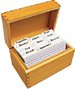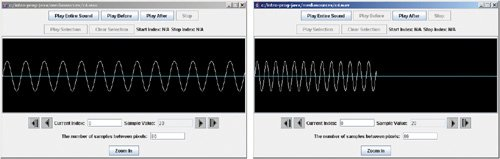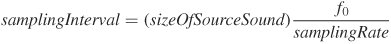# Section 10.4. How Sampling Keyboards Work

[Page 318 (continued)]

### 10.4. How Sampling Keyboards Work

Sampling keyboards are keyboards that use recordings of sounds (e.g., pianos, harps, trumpets) to create music by playing those sound recordings in the desired pitch. Modern music and sound keyboards (and synthesizers) allow musicians to record sounds in their daily lives and turn them into "instruments" by shifting the frequency of the original sounds. How do the synthesizers do it? It's not really complicated. The interesting part is that it allows you to use any sound you want as an instrument.

[Page 319]

Sampling keyboards use huge amounts of memory to record lots of different instruments at different pitches. When you press a key on the keyboard, the recording closest in pitch to the note you pressed is selected, then the recording is shifted to exactly the pitch you requested.

This first method works by creating a sound that skips every other sample. You read that rightafter being so careful to treat all the samples the same, we're now going to skip half of them! In the mediasources directory, you'll find a sound named c4.wav. This is the note C, in the fourth octave of a piano, played for one second. It makes a good sound to experiment with, though really, any sound will work.

##### Program 83. Double the Frequency of a Sound`/** * Method to double the frequency of a sound by taking * every second sample. The result will be a higher * sound. */ public void doubleFreq() { // make a copy of the original sound Sound s = new Sound(this.getFileName()); /* loop through the sound and increment target index * by one but source index by 2 and set target value * to the copy of the original sound */ for (int sourceIndex=0, targetIndex = 0; sourceIndex < this.getLength(); sourceIndex=sourceIndex+2, targetIndex++) this.setSampleValueAt(targetIndex, s.getSampleValueAt(sourceIndex)); // clear out the rest of this sound for (int i = this.getLength() / 2; i < this.getLength(); i++) this.setSampleValueAt(i,0); }`

Here's how to use the double frequency method (Figure 10.4).

`> Sound s = new Sound(FileChooser.getMediaPath("c4.wav")); > s.explore(); > s.doubleFreq(); > s.explore();`

##### Figure 10.4. The original sound (left), and the sound with the frequency doubled (right). (This item is displayed on page 320 in the print version)This method starts like the other ones in this chapter by making a copy of the sound. Then it loops through the sound but it increments the index that keeps the position in the source sound sourceIndex by 2 and the index that keeps the position in the target sound targetIndex by 1. This will copy the sample value at sourceIndex 0 to targetIndex 0, then sourceIndex 2 to targetIndex 1, then sourceIndex 4 to targetIndex 2, and so on. Since the resulting sound will be half as long as it was the second loop just fills the rest of the sound with zeroes.

[Page 320]

Try it! You'll see that the sound really does double in frequency with the result that it sounds higher!

 You are now trying this out as you read, aren't you?

How did that happen? It's not really all that complicated. Think of it like this: the frequency of the original sound is really the number of cycles that pass by in a certain amount of time. If you skip every other sample, the new sound has just as many cycles, but has them in half the amount of time!

Now let's try the other way: Let's take every sample twice! What happens then? To do this, we need to use a cast to "throw away" the fractional part of a floating point number using a cast to integer. To cast a floating point number to an integer number, use (int).

`> System.out.println((int)0.5) 0 > System.out.println((int)1.5) 1`

Here's the method that halves the frequency. The for loop moves the value of the variable targetIndex along the length of the sound. The sourceIndex is now being incrementedbut only by 0.5! The effect is that we'll take every sample in the source twice. The sourceIndex will be 0.0, 0.5, 1.0, 1.5, and so on, but because we're using the (int) of that value, we'll take samples 0, 0, 1, 1, and so on.

##### Program 84. Half the Frequency (This item is displayed on pages 320 - 321 in the print version)`/** * Method to halve the frequency of a sound by taking * each sample twice. The result will be a lower * sound. */ [Page 321] public void halveFreq() { // make a copy of the original sound Sound s = new Sound(this.getFileName()); /* loop through the sound and increment target index * by one but source index by 0.5 and set target value * to the copy of the original sound */ for (double sourceIndex=0, targetIndex = 0; targetIndex < this.getLength(); sourceIndex=sourceIndex+0.5, targetIndex++) this.setSampleValueAt((int) targetIndex, s.getSampleValueAt((int) sourceIndex)); }`

This method first creates a copy of the sound. Then it loops through the sound incrementing the sourceIndex by 0.5 and the targetIndex by 1. We get a sample value from source at the integer value using ((int)) of the sourceIndex. We set the target at the integer value using ((int)) of the targetIndex to the sample value that we got from the copy of the sound. We then add 0.5 to the sourceIndex. This means that the sourceIndex, the first few times through the loop, will take on the values 0.0, 0.5, 1.0, 1.5, 2.0, 2.5, and so on. But the integer part of this sequence is 0, 0, 1, 1, 2, 2, and so on. The result is that we take each sample from the source sound twice.

Think about what we're doing here. Imagine that the 0.5 above were actually 0.75 or 3.0. Would this work? The for loop would have to change, but essentially the idea is the same in all these cases. We are sampling the source data to create the target data. Using a sample index of 0.5 slows down the sound and halves the frequency. A sample index larger than one speeds up the sound and increases the frequency.

Let's try to generalize this sampling with the method below. (Note that this one won't work right!)

##### Program 85. Changing the Frequency of a Sound: BROKEN! (This item is displayed on pages 321 - 322 in the print version)`/** * Method to change the frequency of a sound by the * passed factor * @param factor the amount to increment the source * index by. A number greater than 1 will increase the * frequency and make the sound higher * while a number less than one will decrease the * frequency and make the sound lower. */ public void changeFreq(double factor) { // make a copy of the original sound [Page 322] Sound s = new Sound(this.getFileName()); /* loop through the sound and increment the target index * by one but increment the source index by the factor */ for (double sourceIndex=0, targetIndex = 0; targetIndex < this.getLength(); sourceIndex=sourceIndex+factor, targetIndex++) { this.setSampleValueAt((int) targetIndex, s.getSampleValueAt((int) sourceIndex)); } }`

Here's how we could use this:

`> s = new Sound(FileChooser.getMediaPath("c4.wav")); > s.explore(); > s.changeFreq(0.75); > s.explore();`

That will work really well! But what if the factor for sampling is MORE than 1.0?

`> String fileName = FileChooser.getMediaPath("Elliot-hello.wav"); > Sound hello = new Sound(fileName); > hello.changeFreq(1.5); You are trying to access the sample at index: 54759, but the last valid index is at 54757.`

Why? What's happening? Here's how you could see it: Print out the sourceIndex just before the setSampleValueAt. You'd see that the sourceIndex becomes larger than the source sound! Of course, that makes sense. If each time through the loop, we increment the targetIndex by 1, but we're incrementing the sourceIndex by more than one, we'll get past the end of the source sound before we reach the end of the target sound. But how do we avoid it?

Here's what we want to happen: If the sourceIndex ever gets equal to or larger than the length of the source, we want to reset the sourceIndexprobably back to 0. The key word here is if.

As you may recall from Section 6.1, we can tell Java to make decisions based on a test. We use an if statement to execute a group of statements if a test evaluates to true. In this case, the test is sourceIndex >= s.getLength(). We can test on <, >, == (for equality), != (for inequality, not-equals) and even <= and >=. An if statement can take a block of statements, just as while and for do. The block defines the statements to execute if the test in the if statement is true. In this case, our block is simply sourceIndex = 0;. The block of statements is defined inside of an open curly brace '{' and a close curly brace '}'. If you just have one statement that you want to execute, it doesn't have to be in a block, but it is better to keep it in a block.

[Page 323]

The method below generalizes this and allows you to specify how much to shift the samples by.

##### Program 86. Changing the Frequency of a Sound`/** * Method to change the frequency of a sound * by the passed factor * @param factor the amount to increment the source * index by. A number greater than 1 will increase the * frequency and make the sound higher * while a number less than one will decrease the frequency * and make the sound lower. */ public void changeFreq2(double factor) { // make a copy of the original sound Sound s = new Sound(this.getFileName()); /* loop through the sound and increment the target index * by one but increment the source index by the factor */ for (double sourceIndex=0, targetIndex = 0; targetIndex < this.getLength(); sourceIndex=sourceIndex+factor, targetIndex++) { if (sourceIndex >= s.getLength()) { sourceIndex = 0; } this.setSampleValueAt((int) targetIndex, s.getSampleValueAt((int) sourceIndex)); } }`

We can actually set the factor so that we get whatever frequency we want. We call this factor the sampling interval. For a desired frequency f0, the sampling interval should be:This is how a keyboard synthesizer works. It has recordings of pianos, voices, bells, drums, whatever. By sampling those sounds at different sampling intervals, it can shift the sound to the desired frequency.

The last method of this section plays a single sound at its original frequency, then at two times, three times, four times, and five times the frequency. We need to use blockingPlay to let one sound finish playing before the next one starts. Try it with play and you'll hear the sounds collide as they're generated faster than the computer can play them.

[Page 324]
##### Program 87. Playing a Sound in a Range of Frequencies`/** * Method to play a sound 5 times and each time increase the * frequency. It doesn't change the original sound. */ public void play5Freq() { Sound s = null; // loop 5 times but start with 1 and end at 5 for (int i = 1; i < 6; i++) { // reset the sound s = new Sound(this.getFileName()); // change the frequency s.changeFreq(i); // play the sound s.blockingPlay(); } }`

To use this method, try:

`> Sound s = new Sound(FileChooser.getMediaPath("c4.wav")); > s.play5Freq();`

This method loops with the value of i starting at 1 and ending before it is 6. This will loop five times. Why start at 1 instead of 0? What would happen if we used a factor of 0 to change the frequency? We would end up with silence for the first sound.

#### 10.4.1. Sampling as an Algorithm

You should recognize similarity between the halving recipe (method) Program 84 (page 320) and the recipe for scaling a picture up (larger) Program 31 (page 162). To halve the frequency, we take each sample twice by incrementing the source index by 0.5 and using the casting (int) to get the integer part of that. To make the picture larger, we take each pixel twice, by adding 0.5 to the source index variable and using the casting on that. These two methods are using the same algorithm. The details of pictures vs. sounds aren't critical. The point is that the same basic process is being used in each.

We have seen other algorithms that cross media boundaries. Obviously, our increasing red and increasing volume methods (and the decreasing versions) are essentially doing the same things. The way that we blend pictures or sounds is the same. We take the component color channels (pixels) or samples (sounds) and add them using percentages to determine the amount from each that we want in the final product. As long as the percentages total 100%, we'll get a reasonable output that reflects the input sounds or pictures at the correct percentages.

[Page 325]

Identifying algorithms like these is useful for several reasons. If we understand the algorithm in general (e.g., when it's slow and when it's fast, what it works for and what it doesn't, what the limitations are), then the lessons learned apply in the specific picture or sound instances. The algorithms are also useful for designers to know. When you are designing a new program, you can keep in mind the algorithms that you know so that you can use them when they apply.

When we double or halve the sound frequency, we are also shrinking and doubling the length of the sound (respectively). You might want a target sound whose length is exactly the length of the sound, rather than have to clear out extra stuff from a longer sound. You can do that with new Sound(int lengthInSamples). new Sound(44000) returns a new empty sound of 44,000 samples.Introduction to Computing & Programming Algebra in Java(c) A Multimedia Approach
ISBN: N/A
EAN: N/A
Year: 2007
Pages: 191

flylib.com © 2008-2017.
If you may any questions please contact us: flylib@qtcs.net